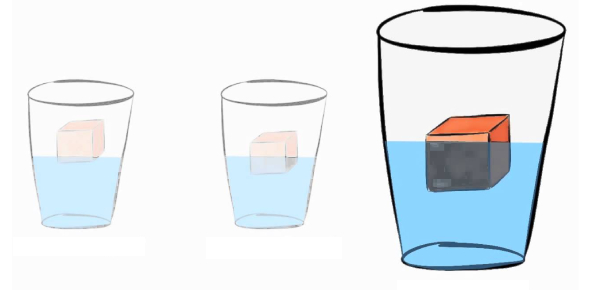# Concept Of Density Quiz Questiosn

8 Questions | Attempts: 9243Settings.

• 1.
What is the density of an object with a mass of 48 grams and a volume of 3 ml?
• A.

144 g/ml

• B.

16 g/ml

• C.

45 g/ml

• D.

51 g/ml

• 2.
A 10 cm3 sample of metal has a mass of about 90 g. What is the density of the metal?
• A.

9 g/cm3

• B.

900 g/cm3

• C.

80 g/cm3

• D.

100 g/cm3

• 3.
A piece of Silver has a mass of 77 grams and a volume of 11 cm3.  What is the density of Silver?
• A.

88 g/cm3

• B.

7 g/cm3

• C.

77 grams

• D.

11 cm3

• 4.
Two cubes of the same size were measured.  Both cubes had the same volume (size).  Cube A was 52 grams and cube B was 130 grams.  Which cube has the greatest density?   Cube A   52 g Cube B   130 g
• A.

Cube A

• B.

Cube B

• C.

Option 3

• D.

Option 4

• 5.
Orange colored block 54 grams 9 cm3 clear liquid 100 grams 100 ml Steel cube 81 grams 9 cm3 piece of aluminum 50 grams 25 cm3 Which object has the lowest density?
• A.

Aluminum

• B.

Steel

• C.

Clear liquid

• D.

Orange colored block

• 6.
What is the volume of a rectangular shaped object with a length of 5 cm, a width of 2 cm, and a height of 10 cm? (Hint: volume of rectangular solid= l  x  w   x   h)
• A.

17 cm3

• B.

100 cm3

• C.

3 cm3

• D.

200 cm3

• 7.
The round object was placed in water to determine its volume.  Before it was placed in the water, there was 100 ml of water.  After it was submerged, 30 ml of water flowed out to the smaller container.  What is the volume of the round object?
• A.

30 ml

• B.

100 ml

• 8.
A 48 gram metal bar was placed in water.  The initial water level was 80 ml.  The final volume was 86 ml.  What is the volume of the bar and its density?
• A.

Volume= 6 ml, density= 8 g/ml

• B.

Volume= 6 ml, density = 54 g/ml

• C.

Volume= 6 ml, density= 180 g/ml

• D.

Volume = 6 ml, density= 0 g/ml

## Related TopicsBack to top
×

Wait!
Here's an interesting quiz for you.